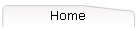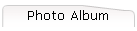#### 10/15/05## SUMMARY OF RESEARCH ACCOMPLISHMENTS

Though originally developed as a natural outgrowth of multivariable calculus, algebraic topology and differential forms have become an essential tool used to formulate many basic laws of physics. Through my research this area of mathematics has found a natural application to many areas of electrical engineering and computational electromagnetics. A strong theme in my research is the identification of geometric and topological aspects, which shed light on dimensional dependence in the complexity of engineering problems and their algorithmic solution. Specifically, (numbers refer to publications list, see also “other significant conference papers”)

·        Cohomology theory and 3-D boundary value problems ,,,Techniques from algebraic and differential topology are used in the formulation of variational boundary value problems and in the development of algorithms for handling topological constraints in numerical solutions.  These techniques are essential to the efficient solution of magnetostatic and eddy current problems in three-dimensions. In this area Hodge theory for manifolds with boundary gives the right framework for investigating the global aspects of gauge transformations and conservation laws for both primal and dual variational principles associated with a given boundary value problem.

·        Data Structures for Finite Element Analysis ,, Results on data structures for the finite element analysis of three dimensional electromagnetic fields have come about as a byproduct of applying topological tools to what I thought were more challenging problems. First, Whitney forms were mentioned in my Ph.D. thesis as the obvious way of reconciling Maxwell’s equations with simplicial data structures. It was only through the heroic efforts of Alain Bossavit and the work at EDF that this point of view has become widely accepted. Second, in the process of freeing the computation of homology groups of floating-point arithmetic, my algorithms for the computation of cuts for scalar potentials were rooted in the notion of a simplicial complex. This data structure is quickly becoming a basic tool in the field and the implicit homotopical information is leading to some very surprising new developments. Third, the Whitney form dicretization of helicity functionals has lead to unexpected ties to basic three- dimensional manifold invariants through the formalism of quantum field theory. The irony is that in all of these cases, the data structures have gained wider acceptance than the concrete problems where I introduced them as essential tools.

·        Cuts for magnetic scalar potentials ,,,,,,,Cohomology theory is exploited in the scalar potential formulation of quasistatic magnetic field problems involving multiply connected current free regions. Specifically, torsion information in integral cohomology groups yields the information required for coupling magnetic scalar potentials to stream functions describing surface currents. Level sets of harmonic maps into the circle (the Eilenberg-MacLane space K(Z,1)) play a key role in representing “cuts” for scalar potentials in terms of embedded manifolds. Cohomology operations (Bockstein homomorphism and Massey products) yield many intuitive insights into the topological structure of 3-D boundary value problems. All of the above aspects can be treated systematically and algorithmically in the context of the finite element method by appealing to simplicial cohomology theory. Our work shows that beyond the work required to solve Poisson's equation, an O(n4/3) time and O(n2) storage algorithm can always be implemented. When heuristic assumptions are made about the region, (the exact consequences of which are being explored), we have implemented an O(n) storage algorithm which requires the solution of Poisson's equation and an additional O(n2) time. This work enables a speed up of a factor of 7--9 for solving time varying problems (for example:  eddy current nondestructive testing) and nonlinear problems such as magnet and motor design. Recently, this work has opened the door to examining the role of knotted current paths in device design.

·        Helicity functionals in compuational electromagnetics ,, ,   The metric independence of helicity invariants has been identified as being very useful in the finite element formulation of certain 3-D boundary value problems of technological importance.  The use of a helicity functional leads to smaller condition numbers for resulting finite element matrices as well as a simpler matrix assembly and numerical methods.  These techniques are presently being developed in the context of nonlinear and inverse problems.

Here, a mathematical remark is in order.  Early in the investigation of Whitney form based finite element analysis of helicity functionals, I developed a discrete version of metric invariance at about the same time as Ed Witten developed a quantum field theoretic view of the Jones polynomial.  The latter has created a lot of interest in “Abelian Chern-Simmons Theory” which, being related to the helicity functional, is enriched by my work on Whitney form discretizations. Specifically, both Werner Muller's proof of the Ray-Singer conjecture (equating analytic torsion with Reidemeister torsion,) and Albert Schwartz's reinterpretation of the conjecture in terms of quantum field theoretic partition functions, can be made very concrete by appealing to my Whitney form discretizations of helicity functionals.  On manifolds with boundary, the partition function is no longer Reidemeister torsion and hence no longer a topological invariant.  Instead, it is a product of a topological term and the determinant of a Dirichlet to Neumann map. This and other aspects of Whitney form discretizations of helicity functionals yield geometric insights into three-dimensional impedance tomography.

·        Micromagnetics , , , , ,  In the area of micromagnetics, the investigation of Bloch points and their effect on vertical Bloch line memories requires a 3-D formulation which does not make use of the usual ``thin film'' assumptions.  Here the use of obstruction theory from algebraic topology naturally points to the important role played by the degree of a mapping between compact two-dimensional manifolds. The expression of this winding number as an integral offers insight into Bloch points both on the conceptual level and in the context of numerical simulations. These techniques are similar to those encountered in condensed matter physics and the classification of defects in ordered media.  By pursuing the use of topological methods, a complete understanding of the "topological accessibility" problem has been obtained by appealing theory underlying the Hopf invariant of algebraic topology. Here one wants to know whether one micromagnetic configuration can be deformed into another in a continuous fashion. These topological conservation laws developed for micromagnetics have been considered in the context of reversible computation with micromagnetic structures.

·        Arithmetical Topology for the Analysis of Algorithms ,   Simple considerations of combinatorial topology enable one to estimate the number of degrees of freedom and the number of nonzero entries in a finite element stiffness matrix arising from an unstructured mesh. Such an analysis enables one to derive exact formulas for the number of FLOPS/CG iteration and evaluate the relative merits of different finite element formulations of three dimensional boundary value problems for vector fields (e.g. tetrahedral vs. hexahedral element; node vs. edge based elements). These techniques are used to articulate why direct solvers such as Gaussian elimination are often used for accurate two- dimensional analyses, but semi-direct algorithms such as conjugate gradients are indispensible for three-dimensional problems. These analyses are also applicable to solution strategies involving h and p refinement on both serial and massively parallel computers

Home | Research | Publications | Bookshelf | Photo Album | Teaching | Links

This site was last updated 10/15/05